﻿ 基于Spark计算框架的分布式文本分类方法研究 A Comparative Study of Distributed Text Categorization Methods Based on Different Computing Frameworks

Hans Journal of Data Mining
Vol. 08  No. 04 ( 2018 ), Article ID: 26723 , 11 pages
10.12677/HJDM.2018.84017

A Comparative Study of Distributed Text Categorization Methods Based on Different Computing Frameworks

Huifeng Tang1, Wei Guo2, Le Zhang2

1Information Engineering University of Strategic Support Force, Zhengzhou Henan

2Graduate School, Information Engineering University of Strategic Support Force, Zhengzhou Henan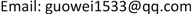Received: Aug. 15th, 2018; accepted: Aug. 30th, 2018; published: Sep. 6th, 2018ABSTRACT

In view of the low efficiency of traditional text classification algorithms in the face of increasing mass of text data, a parallel naive Bayes text classifier is designed and implemented on the Spark computing framework, and the implementation process of text classification based on Spark computing framework is introduced. In the experimental stage, the efficiency of text classification is tested under three different computing frameworks, single machine, Map Reduce and Spark, and control experiments are designed under the Spark computing framework using control variables. Experiments show that naive Bayes algorithm in Spark computing framework has high efficiency in dealing with massive text categorization.

Keywords:Text Classification, Computational Framework, Naive Bayes, TF-IDF

1战略支援部队信息工程大学，河南 郑州

2战略支援部队信息工程大学研究生院，河南 郑州1. 引言

2. 相关技术发展现状

2.1. 文本分类技术

$\phi :D\to C$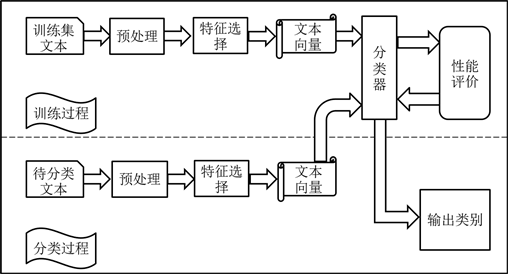Figure 1. Text classification process

2.2. 分布式计算框架

Map Reduce计算框架的核心思想是“分而治之”，就是把较大的任务分解成若干小任务，然后分发到集群的各个计算节点上分别进行计算，最后将结果汇总。整个过程可分为Map和Reduce两个阶段。在Map阶段会将待处理的原始数据按照一定原则分割成数据块，然后分发到各个计算节点上进行计算；Reduce阶段会把Map阶段得到的结果进行汇总，合并成最终的结果。

2.2.2. Spark计算框架

• 中间输出和结果存储在内存中，不再需要读写HDFS，极大地提高了任务的执行速度。

• 使用RDD (Resilient Distributed Datasets)作为分布式索引来对数据进行分区和处理，能够减少机器之间的数据混合(Data Shuffling)。

• Map Reduce在数据Shuffle时每次都花费大量时间来排序，而Spark任务在Shuffle中不是所有情况都需要排序。

3. 文本分类算法流程

3.1. 文本预处理

3.1.1. 分词

3.1.2. 去除停用词

3.2. 文本特征选择

3.3. 文本表示

$T{F}_{{w}_{ij}}=\frac{{n}_{{w}_{ij}}}{\sum _{k}{n}_{{w}_{kj}}}$

IDF (Inverse Document Frequency)为特征项的逆向文本频率，反映了特征词在整个文本集合的分布情况。其定义如下：

$ID{F}_{{w}_{i}}=\mathrm{log}\frac{M}{\sum _{j}|j:{w}_{i}\in {d}_{j}|}$

$TFID{F}_{{w}_{ij}}=T{F}_{{w}_{ij}}×ID{F}_{{w}_{ij}}$

3.4. 分类方法选择

$P\left({C}_{i}|X\right)=\frac{P\left(X|{C}_{i}\right)P\left({C}_{i}\right)}{P\left(X\right)}$

$P\left({C}_{i}\right)={n}_{i}/N$

$P\left(X|{C}_{i}\right)=P\left({X}_{1}|{C}_{i}\right)P\left({X}_{2}|{C}_{i}\right)\cdots P\left({X}_{n}|{C}_{i}\right)=\prod _{j=1}^{n}P\left({X}_{j}|{C}_{i}\right)$

$P\left({X}_{j}|{C}_{i}\right)=\frac{1+TF\left({X}_{j},{C}_{i}\right)}{|V|+\prod _{j=1}^{|V|}TF\left({X}_{j},{C}_{i}\right)}$

4. 基于Spark的朴素贝叶斯算法设计与实现

4.1. 数据预处理

• 通过读取目录的方式将文本集读入，分别用不同的int型数值作为每一类的类标号label；

• 利用中文分词工具对文本集进行分词；

• 按照贝叶斯模型的输入要求转换输入格式：每个类别形成一个新文本，新文本中每行存放一篇文本，每篇文本的格式为 ，即类别名和文档分词后的词组成的集合；

• 预处理完成后，会在新目录transformed Train下生成与类别数相同的多个文本，这些文本以类标号label命名，如0.txt~19.txt。将预处理过后的数据上传至HDFS中。

4.2. 文本向量化

$IDF\left(t,D\right)=\mathrm{log}\frac{|D|+1}{DF\left(t,D\right)+1}$

TF-IDF度量值表示如下：

$TFIDF\left(t,d,D\right)=TF\left(t,d\right)\cdot IDF\left(t,D\right)$

TF：将输入的文本转换成固定长度的特征向量，通过应用hash函数将原始特征映射到index，即index就是单词的哈希值，value是根据映射的index计算的单词频数。

IDF：作用于一个数据集并产生一个IDF模型，该IDF模型接收由上一步产生的特征向量，计算每个词在语料库中出现的文本数目。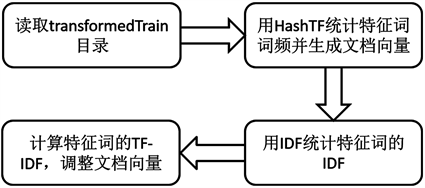Figure 2. Flow chart of text Vectorization

4.3. 模型训练

Spark MLlib模型训练的输入数据的格式是RDD(label,features)。训练完成后，生成朴素贝叶斯分类模型。具体步骤如下：

• 准备训练数据trainDataRdd，训练数据的格式是RDD(label,features)；

• 调用类伴生对象NaiveBayes的静态train方法，根据输入参数，初始化NaiveBayes类，然后开始训练模型。训练完成后，生成一个贝叶斯分类模型。

5. 实验与分析

5.1. 实验环境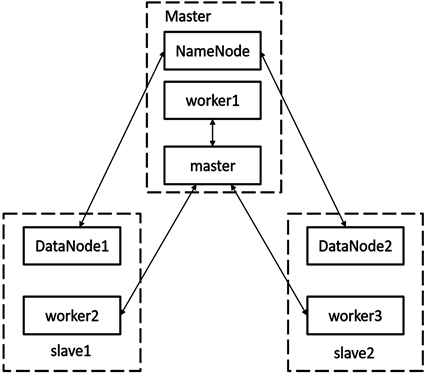Figure 3. Node deployment schematic

5.2. 实验语料

5.3. 实验设计及结果分析

5.3.1. 实验一

• 单机环境

• Map Reduce计算框架

• Spark计算框架

• 结果分析Table 3. Experimental results under single machine environmentTable 4. Experimental results in Hadoop map reduce environmentTable 5. Experimental results under spark environment

5.3.2. 实验二

5.3.3. 实验三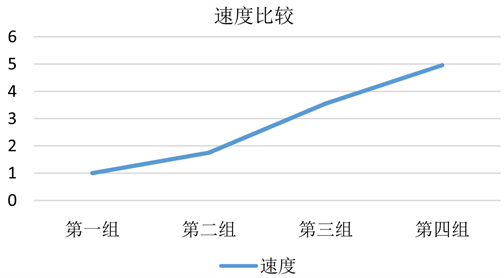Figure 4. The influence of the number of calculated nodes on the speed of operationTable 6. The average running time of the three groups of experiments

6. 总结

A Comparative Study of Distributed Text Categorization Methods Based on Different Computing Frameworks[J]. 数据挖掘, 2018, 08(04): 151-161. https://doi.org/10.12677/HJDM.2018.84017

1. 1. 罗元帅. 基于随机森林和Spark的并行文本分类算法研究[D]: [硕士学位论文]. 成都: 西南交通大学, 2016.

2. 2. Ghemawat, S., Gobioff, H. and Leung, S. (2003) File and Storage Systems: The Google File System. ACM Symposium on Operating Systems Principles, Bolton Landing, 19-22 October 2003, Vol. 37, 29-43. https://doi.org/10.1145/1165389.945450

3. 3. Dean, J. and Ghemawat, S. (2004) Map Reduce: Simplified Data Processing on Large Clusters. Proceedings of Operating Systems Design and Implementation (OSDI), 51, 107-113.

4. 4. Chang, F., Dean, J., Ghemawat, S., et al. (2006) Big Table: A Distributed Storage System for Structured Data. Proceedings of Use Nix Symposium on Operating Systems Design & Implementation, 26, 205-218.

5. 5. 光顺利. 基于Spark的文本分类的研究[D]: [硕士学位论文]. 长春: 长春工业大学, 2016.

6. 6. Luhn, H.P. (1959) Au-to-Encoding of Documents for Information Retrieval Systems. Modem Trends in Documentation. Pergamon Press, New York.

7. 7. 侯汉清. 分类法的发展趋势简论[M]. 北京: 中国人民出版社, 1981.

8. 8. Yang, Y. (1999) An Evaluation of Statistical Approaches to Text Categorization. Information Retrieval, 1, 69-90. https://doi.org/10.1023/A:1009982220290

9. 9. 庞剑锋, 卜东波. 基于向量空间模型的文本自动分类系统的研究与实现[J]. 计算机应用研究, 2001, 18(9): 23-26.

10. 10. 张志飞, 苗夺谦, 高灿. 基于LDA主题模型的短文本分类方法[J]. 计算机应用, 2013, 33(6): 1587-1590.

11. 11. 武建军, 李昌兵. 基于互信息的加权朴素贝叶斯文本分类算法[J]. 计算机系统应用, 2017, 26(7): 178-182.

12. 12. 江小平, 李成华, 向文, 张新访. 云计算环境下朴素贝叶斯文本分类算法的实现[J]. 计算机应用, 2011, 31(9): 2551-2554.

13. 13. 宋福星. 基于Spark的超大文本分类方法的设计与实现[D]: [硕士学位论文]. 北京: 北京交通大学, 2017.

14. 14. 贺鸣, 孙建军, 成颖. 基于朴素贝叶斯的文本分类研究综述[J]. 情报科学, 2016, 34(7): 147-154.

15. 15. 李方, 刘琼荪. 基于改进属性加权的朴素贝叶斯分类模型[J]. 计算机工程与应用, 2010, 46(4): 132-133.